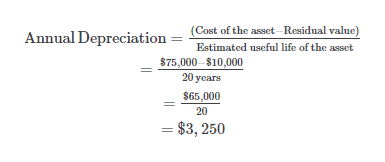# A storage tank acquired at the beginning of the fiscal year at a cost of \$75,000 has an estimated residual value of \$10,000 and an estimated useful life of 20 years. Determine the following: (a) the amount of annual depreciation by the straight-line method and (b) the amount of depreciation for the first and second years computed by the doubledeclining-balance method.

Question
1 views

A storage tank acquired at the beginning of the fiscal year at a cost of \$75,000 has an estimated residual value of \$10,000 and an estimated useful life of 20 years. Determine the following: (a) the amount of annual depreciation by the straight-line method and (b) the amount of depreciation for the first and second years computed by the doubledeclining-balance method.

check_circle

Step 1

(a)

Calculate  the amount of annual deprec...help_outlineImage Transcriptionclose(Cost of the asset-Residual value) Annual Depreciation Estimated useful life of the asset \$75,000–\$10,000 20 years \$65,000 20 = \$3, 250 fullscreen

### Want to see the full answer?

See Solution

#### Want to see this answer and more?

Solutions are written by subject experts who are available 24/7. Questions are typically answered within 1 hour.*

See Solution
*Response times may vary by subject and question.
Tagged in